## Example 80.1 Creating Fixed-Sample Designs

This example demonstrates a one-sided fixed-sample design and a two-sided fixed-sample design. The following statements request a fixed-sample design with an upper alternative:

```ods graphics on;
proc seqdesign pss
;
OneSidedFixedSample: design nstages=1
alt=upper
alpha=0.025 beta=0.10
;
samplesize model=onesamplemean(mean=0.25);
run;
ods graphics off;
```

In the DESIGN statement, the label OneSidedFixedSample identifies the design in the output tables. The NSTAGES=option specifies that the design has only one stage; this corresponds to a fixed-sample design. In the SEQDESIGN procedure, the null hypothesis for the design is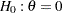and the ALT=UPPER option specifies an upper alternative hypothesis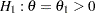. The MEAN=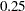option in the SAMPLESIZE statement specifies the upper alternative reference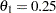.

The options ALPHA=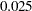and BETA=
specify the Type I error probability level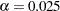and the Type II error probability level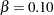. That is, the design has a power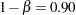at.

The "Design Information" table in Output 80.1.1 displays design specifications and the derived statistics such as power. As expected, the derived statistics such as maximum information and average sample number (in percentage of its corresponding fixed-sample information) are
for the fixed-sample design (NSTAGES=1). Also, for a fixed-sample design, the STOP= and METHOD= options in the DESIGN statement are not applicable.

The SEQDESIGN Procedure
Design: OneSidedFixedSample

Statistic Distribution Normal
Boundary Scale Standardized Z
Alternative Hypothesis Upper
Alternative Reference 0.25
Number of Stages 1
Alpha 0.025
Beta 0.1
Power 0.9
Max Information (Percent of Fixed Sample) 100
Max Information 168.1188
Null Ref ASN (Percent of Fixed Sample) 100
Alt Ref ASN (Percent of Fixed Sample) 100

The "Method Information" table in Output 80.1.2 displays theanderror levels. It also displays the derived drift parameter, which is the standardized reference improvement,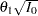, where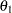is the alternative reference and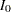is the maximum information for the design. If eitheroris specified, the other statistic is derived in the SEQDESIGN procedure. For a fixed-sample design,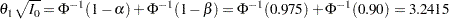Output 80.1.2 Method Information
Method Information
Boundary Alpha Beta Alternative
Reference
Drift
Upper Alpha 0.02500 0.10000 0.25 3.241516

The "Boundary Information" table in Output 80.1.3 displays information level, alternative reference, and boundary value at each stage. The information proportion indicates the proportion of maximum information available at the stage. With only one stage for a fixed-sample design, the proportion is. With the SAMPLESIZE statement, the required sample size
is also displayed under the heading "Information Level."

Output 80.1.3 Boundary Information
Boundary Information (Standardized Z Scale)
Null Reference = 0
_Stage_   Alternative Boundary Values
Information Level Reference Upper
Proportion Actual N Upper Alpha
1 1.0000 168.1188 168.1188 3.24152 1.95996

By default (or equivalently if you specify BOUNDARYSCALE=STDZ), output alternative references and boundaries are displayed with the standardized normal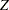scale. The alternative reference on the standardizedscale at stageis given by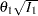, where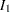is the information level at stage. With a boundary value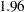, the hypothesis of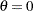is rejected if the standardized normal statistic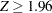.

With ODS Graphics enabled, a detailed boundary plot with the rejection and acceptance regions is displayed, as shown in Output 80.1.4. The boundary values in the "Boundary Information" table in Figure 80.1.3 are displayed in the plot.

Output 80.1.4 Boundary Plot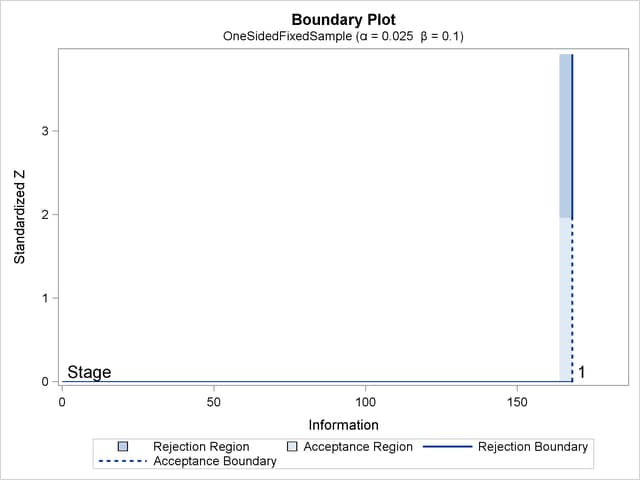The "Sample Size Summary" table in Output 80.1.5 displays parameters for the sample size computation of the test for a normal mean.

Output 80.1.5 Sample Size Summary
Sample Size Summary
Test One-Sample Mean
Mean 0.25
Standard Deviation 1
Max Sample Size 168.1188
Expected Sample Size (Null Ref) 168.1188
Expected Sample Size (Alt Ref) 168.1188

The "Sample Sizes (N)" table in Output 80.1.6 displays the derived sample sizes, in both fractional and integer numbers. With the resulting integer sample sizes, the corresponding information level is slightly larger than the level from the design. This can increase the power slightly if the integer sample size is used in the trial.

Output 80.1.6 Derived Sample Sizes
Sample Sizes (N)
One-Sample Z Test for Mean
_Stage_ Fractional N Ceiling N
N Information N Information
1 168.12 168.1 169 169.0

The following statements request a two-sided fixed-sample design with a specified alternative reference:

```ods graphics on;
proc seqdesign altref=1.2
pss
;
TwoSidedFixedSample: design nstages=1
alt=twosided
alpha=0.05 beta=0.10
;
samplesize model=twosamplemean(stddev=2 weight=2);
run;
ods graphics off;
```

In the SEQDESIGN procedure, the null hypothesis for the design is. The ALT=TWOSIDED option specifies a two-sided alternative hypothesis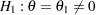. The ALTREF=1.2 option in the PROC SEQDESIGN statement specifies the alternative reference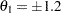.

The ALPHA=0.05 option (which is the default) specifies the two-sided Type I error probability level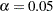. That is, the lower and upper Type I error probabilities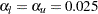. The BETA=0.10 option (which is the default) specifies the Type II error probability level, and the design has a powerat the alternative reference.

The "Design Information" table in Output 80.1.7 displays design specifications and the derived power. With a specified alternative reference, the maximum information is derived.

The SEQDESIGN Procedure
Design: TwoSidedFixedSample

Statistic Distribution Normal
Boundary Scale Standardized Z
Alternative Hypothesis Two-Sided
Alternative Reference 1.2
Number of Stages 1
Alpha 0.05
Beta 0.1
Power 0.9
Max Information (Percent of Fixed Sample) 100
Max Information 7.296822
Null Ref ASN (Percent of Fixed Sample) 100
Alt Ref ASN (Percent of Fixed Sample) 100

The "Method Information" table in Output 80.1.8 displays theanderrors, alternative references, and drift parameter. For a fixed-sample design, the derived drift parameter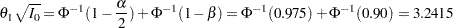Output 80.1.8 Method Information
Method Information
Boundary Alpha Beta Alternative
Reference
Drift
Upper Alpha 0.02500 0.10000 1.2 3.241516
Lower Alpha 0.02500 0.10000 -1.2 -3.24152

With a specified alternative reference, the maximum information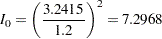The default "Boundary Information" table in Output 80.1.9 displays information level, alternative reference, and boundary values. By default (or equivalently if you specify BOUNDARYSCALE=STDZ), the alternative reference and boundary values are displayed with the standardized normalscale. Thus, the standardized alternative references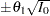are displayed.

Output 80.1.9 Boundary Information
Boundary Information (Standardized Z Scale)
Null Reference = 0
_Stage_   Alternative Boundary Values
Information Level Reference Lower Upper
Proportion Actual N Lower Upper Alpha Alpha
1 1.0000 7.296822 131.3428 -3.24152 3.24152 -1.95996 1.95996

With boundary values of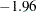and, the hypothesis ofis rejected if the standardized normal statisticor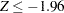.

With ODS Graphics enabled, a detailed boundary plot with the rejection and acceptance regions is displayed, as shown in Output 80.1.10 . The boundary values in the "Boundary Information" table in Figure 80.1.9 are displayed in the plot.

Output 80.1.10 Boundary Plot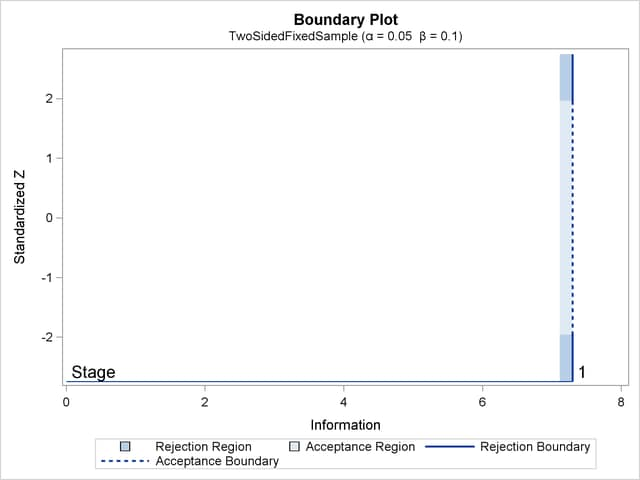The "Sample Size Summary" table in Output 80.1.11 displays parameters for the sample size computation of the test for a normal mean.

Output 80.1.11 Sample Size Summary
Sample Size Summary
Test Two-Sample Means
Mean Difference 1.2
Standard Deviation 2
Max Sample Size 131.3428
Expected Sample Size (Null Ref) 131.3428
Expected Sample Size (Alt Ref) 131.3428
Weight (Group A) 2
Weight (Group B) 1

The "Sample Sizes (N)" table in Output 80.1.12 displays the derived sample sizes, in both fractional and integer numbers. With the WEIGHT=option, the allocation ratio isfor the first group andfor the second group. With the resulting integer sample sizes, the corresponding information level is slightly larger than the level from the design. This can increase the power slightly if the integer sample size is used in the trial.

Output 80.1.12 Derived Sample Sizes
Sample Sizes (N)
Two-Sample Z Test for Mean Difference
_Stage_ Fractional N Ceiling N
N N(Grp 1) N(Grp 2) Information N N(Grp 1) N(Grp 2) Information
1 131.34 87.56 43.78 7.2968 132 88 44 7.3333